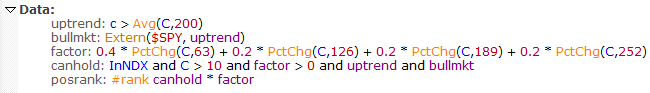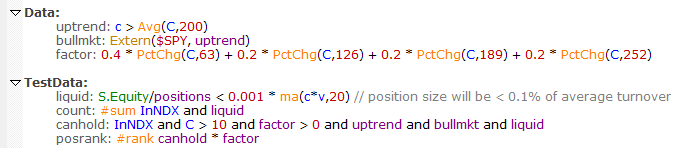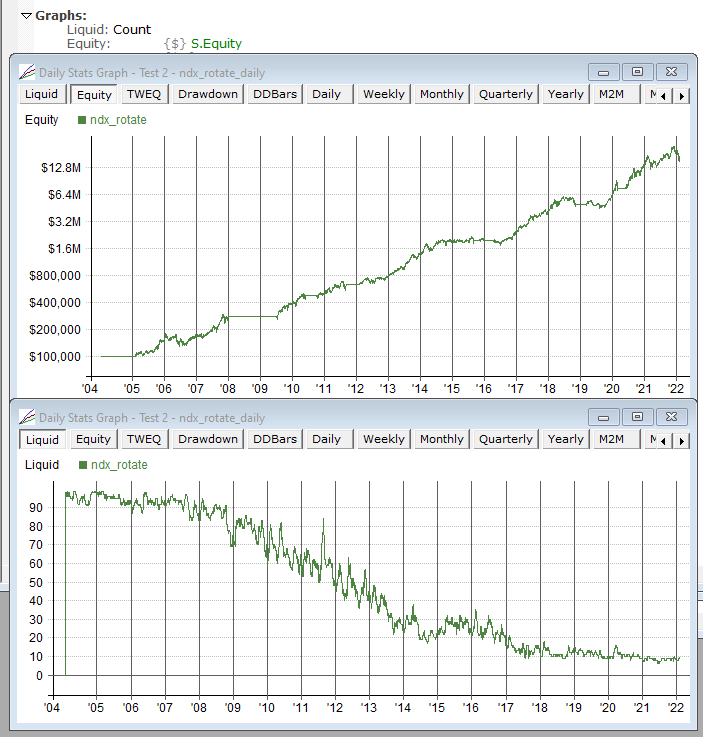Navigation: Realtest Script Language > Script Sections > TestData Section

TestData is a specialized subset and variation of the Data Section.

To summarize their similarities and differences:

 Data Section TestData Section When is it calculated? before any test starts, for all bars of all stocks during each test, for one bar of all stocks at the start of each test date How is it calculated? optimized for speed using multiple threads, one-pass for many indicators, and only recalculating formulas that changed since last time single-threaded, can't use one-pass, all items recalculated every test, but still quite fast if long-looback indicators are avoided What can its formulas reference? bar data, Parameters, previously calculated Data items (including itself), or Library items that only reference these things everything that Data formulas can reference, anything that Strategy formulas can reference, and other TestData items (including itself) Cross-sectional (breadth) support? yes yes How is it stored? in allocated memory arrays: 8 bytes per item * number of stocks * number of bars ditto

Your TestData formulas are evaluated during each test at the start of processing for each test date (BarSize period). At each such interval, they are evaluated for only the current bar of every stock that has a bar for that date, with the value then stored in the TestData item's memory array.

As with Data items, TestData items can be referenced by name for the current bar, any past bar (using [n] offset notation) or as parameters to any multi-bar indicator.

TestData items can also refer to themselves in the same way Data items can.

As an example of how TestData is useful, consider a strategy like the ndx_rotate.rts example where you want to incorporate a liquidity filter based on current S.Equity (account balance) as the test proceeds (assuming no withdrawals).

This example uses the #rank breadth operator to calculate its posrank variable:However, since Data is calculated before the test starts, you can't refer to S.Equity in that formula.

The solution is to move the last two Data items into a TestData section, and add a liquidity calculation:Note that in the above example, S.Equity is referenced directly. This works because there's only one strategy in the script.

A key point to remember, however, is that TestData is not strategy-specific. It is similar to TestScan in this regard. References to test stats, position information, etc. each have an implied Combined wrapper, i.e., the above S.Equity is interpreted as Combined(S.Equity).

If this script contained other strategies, and you wanted to use only this strategy's equity value for your liquidity constraint, you would need to change the above to Extern(@ndx_rotate, S.Equity).

Notice that we've also added a count item, to make it easier to see the impact over time of this liquidity constraint.

By temporarily adding a Graphs item that displays count, we can see at a glance what the impact of this liquidity constraint would have been in this model:In a \$3M+ account, if you applied this constraint, the "Nasdaq 100" would become the "Nasdaq 10" ...